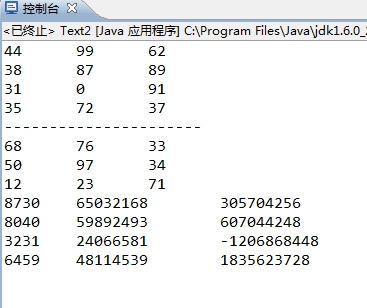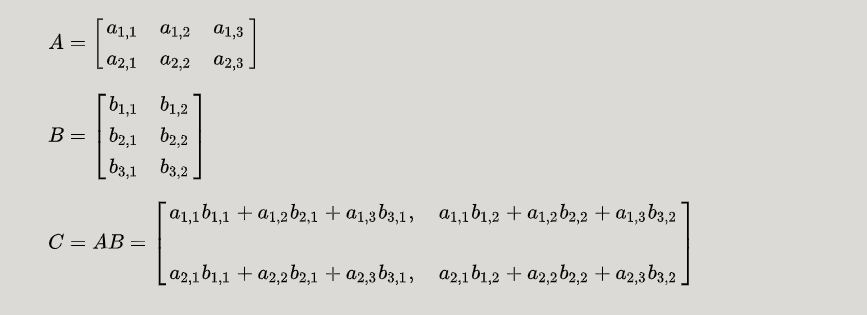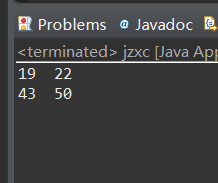• 2022-04-26 01:48:33

import java.io.InputStream;
import java.util.Scanner;
public class Main {
public static void main(String[] args) {
Matrix firstMat=Matrix.inputMatrix();
Matrix secondMat=Matrix.inputMatrix();
//display(firstMat.matrix);    display(secondMat.matrix);

Matrix productMat=firstMat.multiply(secondMat);
display(productMat.matrix);
}

//display方法：打印二维数组元素到屏幕
public static void display(int[][] array){
for(int i=0; i<array.length; i++){
for(int j=0; j<array[i].length; j++){
if(j==array[i].length-1) {
System.out.println(array[i][j]);
}
else {
System.out.print(array[i][j]+" ");
}
}
}
}
}
/*
* 编写程序题, 根据要求编写一个Java类，补充到代码中。
定义Matrix(矩阵)类，使用二维数组实现矩阵，实现两个矩阵的乘法。要求如下：

根据程序需求定义构造方法。
inputMatrix方法：从键盘读入一个矩阵，读入行数和列数，创建矩阵，然后读入元素值方法。
multiply方法：两个矩阵相乘，并返回乘积矩阵。
(两个矩阵的乘法：一个m×n的矩阵a（m,n)乘一个n×p的矩阵b（n,p)，会得到一个m×p的矩阵c（m,p)。矩阵的行数和列数自定。）
*/

class Matrix {
int row;
int column;
int[][] matrix;
static Scanner reader = new Scanner(System.in);

public static Matrix inputMatrix() {
Matrix ret = new Matrix();

ret.matrix = new int[ret.row][ret.column];

for(int i = 0; i < ret.row; i++) {
for(int j = 0; j < ret.column; j++) {

}
}
return ret;
}

Matrix multiply(Matrix mat) {
Matrix ret = new Matrix();
ret.row = row;
ret.column = mat.column;
ret.matrix = new int[row][mat.column];
for(int i = 0; i < row; i++) {
for(int j = 0; j < mat.column; j++) {
for(int k = 0; k < mat.row; k++) {
ret.matrix[i][j] += matrix[i][k] * mat.matrix[k][j];
}
}
}
return ret;
}
}

//注意三重循环使用

//很神奇厉害的地方 可以在类的方法种创建类对象

//类对象也可以作为参数  参数可以是一个类  这个参数包含类中所有的实例与方法(？即便是方法未写完？还是说只能用其中的实例  一般应该也只会用到实例)

//Matrix productMat=firstMat.multiply(secondMat); 调用类的方法

//可以有返回类型为类的方法 注意这种方法的写法

更多相关内容
• 展开全部importjava.util.Random;publicclassText2{publicstaticvoidmain(String[]args){Matrixs3=newMatrix(4,3);Matrixs4=newMatrix(3,3);s3.printMatrix();System.out.println("------------------...

展开全部

import java.util.Random;

public class Text2 {

public static void main(String[] args){

Matrix s3 = new Matrix(4, 3);

Matrix s4 = new Matrix(3, 3);

s3.printMatrix();

System.out.println("----------------------");

s4.printMatrix();

s3.mulMatrix(s4);

s3.printMatrix();

}

public static class Matrix {

private int[][] a;

Random random = new Random();

// 构造方法e69da5e887aa3231313335323631343130323136353331333264656665

public Matrix() {

a = new int;

}

public Matrix(int n) {

a = new int[n][n];

for (int i = 0; i

for (int j = 0; j

a[i][j] = random.nextInt(100);

}

}

}

public Matrix(int n, int m) {

a = new int[n][m];

for (int i = 0; i

for (int j = 0; j

a[i][j] = random.nextInt(100);

}

}

}

public int[][] getMatrix() {

return a;

}

// 打印一个矩阵

public void printMatrix() {

for (int i = 0; i

for (int j = 0; j

System.out.print(a[i][j] + "\t");

}

System.out.println();

}

}

// 矩阵相加

public void plusMatrix(Matrix b) {

int[][] c = b.getMatrix();

int n = c.length;

int m = c.length;

if (n != a.length || m != a.length) {

System.out.println("长度不一，不能相加");

} else {

for (int i = 0; i

for (int j = 0; j

a[i][j] = a[i][j] + c[i][j];

}

}

}

}

// 矩阵相乘

public void mulMatrix(Matrix b) {

int[][] c = b.getMatrix();

int n = c.length;

int m = c.length;

if (n != a.length) {

System.out.println("第一个矩阵的列数不等于第二个矩阵的行数，不能进行乘法运算");

} else {

for (int i = 0; i

for (int j = 0; j

for (int k = 0; k

a[i][j] += (a[i][k] * c[k][j]);

}

}

}

}

}

}

}展开全文• 知识回顾 若A为NxM阶矩阵 B为MxP阶矩阵，二者相乘之后的矩阵则为...设进行矩阵乘法的两个矩阵为a 3x3型矩阵和b 3x2型矩阵 我们在运用数学思维计算矩阵乘法时首先计算的便是 z = a*b+a*b

# 知识回顾

若A为NxM阶矩阵 B为MxP阶矩阵，二者相乘之后的矩阵则为NXP阶矩阵
运算过程：# 思路

• 计算并显示出来这个结果 我们则需要 一个计算方法 和 一个显示方法
• 因此主函数可以写的相当之简单 ， 键入想要求解的矩阵的数值 然后调用两种方法即可

## 显示方法

• 只需要循环显示出行列值然后分行即可

## 计算方法

设进行矩阵乘法的两个矩阵为a 3x3型矩阵和b 3x2型矩阵

• 我们在运用数学思维计算矩阵乘法时首先计算的便是
z = a*b+a*b+a*b
转换成代码则可想到 矩阵可以利用二维数组来实现
下标的改变以及算术累加可以用for循环来实现
• 在此之上我们可以再思考
z计算过后我们将会继续计算z 而列下标的改变则可再次利用循环来实现
以此类推
• 矩阵第一行的数值计算过后 我们要转为求解第二行的数值 这时则可再次利用循环来实现行的改变

因此 二维数组实现矩阵乘法的基本思路就是 利用嵌套循环来实现数值的累加计算，矩阵列以及行的变化。

以下为实现代码：

public class Example4_04{
public static void main(String[] args){
int[][] a={{-4 , 5 , -7},
{2, -3 , -4},
{3 , 4 , 5}};
int[][] b={{1 , 2},
{5 , 6},
{-7 , 9},};
int[][] result = mul(a,b);
print(result);
}
//mul返回值的类型是一个二维数组
static int[][] mul(int[][] x , int[][] y)
{
int i , j , k;//循环控制变量
//申请结果数组的空间
//行长度为x数组的行长度，列长度为y数组第一行的行长度
int[][] z=new int[x.length][y.length];
for(i = 0 ; i < x.length ; i++)
{	for(j = 0 ; j < y.length ; j++)
{
z[i][j] = 0;
for(k = 0 ; k < x.length ; k++)
z[i][j] = z[i][j] + x[i][k] * y[k][j];
}
}
return z;
}
static void print(int[][] x){
int i , j;
for(i = 0 ; i < x.length ; i++){
for(j = 0 ; j < x[i].length ; j++)
System.out.printf("%5d",x[i][j]);
System.out.println();
}
}
}


展开全文算法
• 分别定义两个二维数组用来存放两个矩阵，矩阵A的行号和矩阵B的列号决定矩阵C的行列号；矩阵A的列号和矩阵B的行号相同者进行乘法操作。 现在暂且不考虑设置矩阵相乘的规则，就弄个最简单的矩阵相乘 package juzhen...

分别定义两个二维数组用来存放两个矩阵，矩阵A的行号和矩阵B的列号决定矩阵C的行列号；矩阵A的列号和矩阵B的行号相同者进行乘法操作。

现在暂且不考虑设置矩阵相乘的规则，就弄个最简单的矩阵相乘

package juzhen;

public class jzxc {
public static void main(String[] args) {
int[][] A= {{1,2},{3,4}};
int[][] B= {{5,6},{7,8}};

int C[][]=new int[A.length][];

for(int i=0;i<A.length;i++) {
C[i]=new int[A[i].length];
}

for(int i=0;i<A.length;i++) {
for(int j=0;j<A[i].length;j++) {
for(int k=0;k<A.length;k++) {
C[i][j] +=A[i][k]*B[k][j];
}
}
}
for(int i=0;i<C.length;i++) {
for(int j=0;j<C[i].length;j++) {
System.out.print(C[i][j]+"  ");
}
System.out.println();
}
}
}展开全文• import java.util.*; //由于数组长度不确定，因此我用ArrayList实现 public class ArrayMatrix { public static void main(String[] args) { Scanner input = new Scanner(System.in); System.out.pr.list
• 文章目录线性代数中的矩阵相乘定义注意事项代码逻辑分析代码实现运行结果 线性代数中的矩阵相乘 定义 设A为 的矩阵，B为 的矩阵，那么称 的矩阵C为矩阵A与B的乘积，记作 ，其中矩阵C中的第 行第 列元素可以表示为： ...
• 计算两个二维矩阵的和 目的：掌握二维数组的基本使用方法。 要求：定义两个3*3数组，使用静态初始化的方法设置数组的元素值分别为： 1,2,3 4,5,6 7,8,9 和 0, 2, 4 1.1, 4.3, 5.2 1, 4.5, 2.2 要求编写一个类，类的...
• java二维数组的个数Here, We have to find the multiplication of the two matrix by moving forward direction in row major (row by row) order in one matrix and by backward direction in row major order in ...
• 包括基本数据类型和引用数据类型数组长度确定下来就不能更改创建数组对象会在内存中开辟一段连续的空数组的概述数组名下标或者索引元素数组长度数组有序排列的通过下标进行管理数组长度就是元素数组本身属于引用...
• 输入一个 mn的矩阵 和一个np的矩阵，并初始化, 要求打印出两个矩阵的乘积。 package com.homework.homework0715; import java.util.Arrays; /** * @Author kinmon * @Date 2020/7/15 14:44 * @Version 1.0 * ...
• ## 二维数组 矩阵相乘

千次阅读 2016-12-08 21:22:17
两个矩阵相乘，可以用3个二维数组解决。两个存初始值，一个存结果。 #include using namespace std; #define rows 8 #define cols 8 int a[rows][cols]; int b[rows][cols]; int c[rows][cols]; int main() { int ...
• 利用二维数组实现一矩阵类：Matrix。要求提供以下操作：(1)set(introw,intcol,doublevalue)：将第row行第col列的元素赋值为value；(2)get(introw，intcol)：取第row行第col列的元素；(3)width()：返回矩阵的列数；...
• import java.util.Random; import java.util.Scanner; public class 矩阵运算{ public static void main(String args[]){ Random ran=new Random(); int h1 =0; int l1 =0; int h2=0; int l2=0; for(int i = 0;i<...
• 第一行一1 ，第行21 从第三行开始，每一行的第一数和最后一数都是1 每一行的元素数，和行数相同 从第3行开始，每一行的除第1和最后一元素之外的其他元素， 第i行第j列的值 = 第i-1行第j列值 +
• 前言 本篇文章主要总结了矩阵的常见运算，以及Java中利用二维数组进行运算的实现 1.矩阵运算 1.矩阵加法 运算前提：多矩阵的行数和列数相同，我们称它们为同型矩阵，只有是同型矩阵才能进行加减运算。 运算规则：...程序人生 开发语言 算法
• 二维数组简单来说就是一由行和列组成的一表格，也就是数学中的矩阵。我们在这矩阵中访问元素的话，是根据元素的行角标和列角标所确定的。 那么具体是在内存中如何存储的呢？ 无论是二维数组，还是多维数组...
• 一、 数组的创建 首先我们Java虚拟机（JVM）中包含五部分：Java栈，Java堆，PC寄存 器，方法区和本地方法栈 Java栈： 函数定义的基本数据...二维数组 int[] arr={1,2,3,4,5}; int[][] brr={ {1,2} , {3,4} , {5,6} ...
• 使用二维数组实现Matrix(矩阵)： 1. 定义Matrix(矩阵)类，要求如下： a) 变量：matrix(int型二维数组)，row(行数)，column(列数)； b) 方法：实现两个矩阵的乘法，所有方法将返回操作后的结果矩阵。(两个矩阵的...
• java编写的两个矩阵相乘的算法，打包文件，class
• 可以看到intArray.length这样可以是返回高度10的意思，也就是第一列数组的长度，而intArray.length被用来返回宽度，如果想知道数组总元素个数，可以用这个两个相乘。 ...
• 老师让写两个矩阵相乘，写完后发现，自己一行行地输入数据时，能正常运行。 然而直接通过复制粘贴测试用例输入时，经调试发现程序在接收第数组的行数和列数时，没有读到数据，而是停下，等待输入。 自己试...
• 本文实例为大家分享了Java构建乘积数组的具体实现代码，供大家参考，具体内容如下给定一个数组A[0,1,…,n-1]，请构建一个数组B[0,1,…,n-1]，其中B中的元素B[i]=AA…A[i-1]*A[i+1]…*A[n-1]。不能使用除法。...
• 对python产生随机的二维数组实例详解最近找遍了python的各个函数发现无法直接生成随机的二维数组，其中包括random()相关的各种方法，都没有得到想要的结果。最后在一篇博客中受到启发，通过列表解析的方法得到随机的...
• ## 二维数组

千次阅读 2019-08-01 16:27:26
其实二维数组就是一个二维的矩阵，矩阵的行列对应的位置就是二维数组每个元素对应的下标位置 当然，和一维数组一样，它也是存储在堆内存的，并且每个元素都会进行初始化，所以由此可以看出，二维数组额本质就是一...矩阵的转置
• 定义Matrix(矩阵)类，使用二维数组实现矩阵，实现两个矩阵的乘法。要求如下： 4个变量：row(行数)，column(列数), matrix( int型二维数组)，reader( Scanner对象)。 根据程序需求定义构造方法。 inputMatrix方法：...
• 二维数组简单来说就是一由行和列组成的一表格而已，通俗的说就是一矩阵Matrix。 我们在这矩阵中访问元素的话，是根据元素的行角标和列角标所确定的。 二维数组具体是在内存中如何存储的呢？ 无论是二维...
• 本关任务：给定两个矩阵，求它们的乘积。 矩阵乘示例如下： 图1 矩阵乘 从图 1 可以看到，相乘后的矩阵的行数等于第一个矩阵的行数，列数等于第个矩阵的列数。 编程要求 仔细阅读右侧编辑区内给出的...
• 所谓二维数组，就是一由行和列组成的一表格而已，矩阵Matrix。我们在这矩阵中访问元素的话，是根据元素的行角标和列角标所确定的。 那二维数组具体是在内存中如何存储的呢？ 无论是二维数组，还是多维...
• 这道题目的关键是，求列上的数字之和时，保持列不变，行相加；求行上数字之和时，保持行不变，列相加 import java.util.*;...遍历该二维数组 4.保持列不变，遍历行上的数字，让他们相加 */ ......

# 两个二维数组相乘javajava 订阅